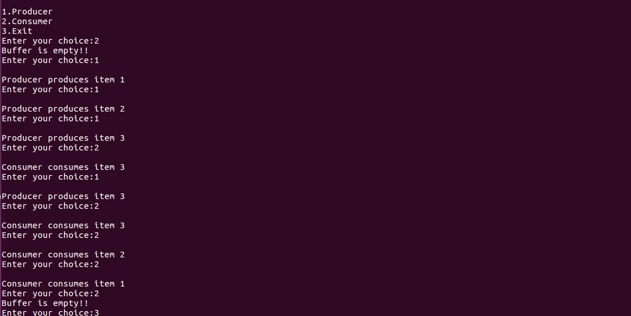# Producer Consumer Problem in C

• Difficulty Level : Hard
• Last Updated : 18 Mar, 2021

The producer-consumer problem is an example of a multi-process synchronization problem. The problem describes two processes, the producer and the consumer that shares a common fixed-size buffer use it as a queue.

• The producer’s job is to generate data, put it into the buffer, and start again.
• At the same time, the consumer is consuming the data (i.e., removing it from the buffer), one piece at a time.

Problem: Given the common fixed-size buffer, the task is to make sure that the producer can’t add data into the buffer when it is full and the consumer can’t remove data from an empty buffer.

We provide nothing but the best curated videos and practice problems for our students. Check out the C Foundation Course and master the C language from basic to advanced level. Wait no more, start learning today!

Solution: The producer is to either go to sleep or discard data if the buffer is full. The next time the consumer removes an item from the buffer, it notifies the producer, who starts to fill the buffer again. In the same manner, the consumer can go to sleep if it finds the buffer to be empty. The next time the producer puts data into the buffer, it wakes up the sleeping consumer.

Note: An inadequate solution could result in a deadlock where both processes are waiting to be awakened.

Approach: The idea is to use the concept of parallel programming and Critical Section to implement the Producer-Consumer problem in C language using OpenMP

Below is the implementation of the above approach:

## C

 `// C program for the above approach`` ` `#include ``#include `` ` `// Initialize a mutex to 1``int` `mutex = 1;`` ` `// Number of full slots as 0``int` `full = 0;`` ` `// Number of empty slots as size``// of buffer``int` `empty = 10, x = 0;`` ` `// Function to produce an item and``// add it to the buffer``void` `producer()``{``    ``// Decrease mutex value by 1``    ``--mutex;`` ` `    ``// Increase the number of full``    ``// slots by 1``    ``++full;`` ` `    ``// Decrease the number of empty``    ``// slots by 1``    ``--empty;`` ` `    ``// Item produced``    ``x++;``    ``printf``(``"\nProducer produces"``           ``"item %d"``,``           ``x);`` ` `    ``// Increase mutex value by 1``    ``++mutex;``}`` ` `// Function to consume an item and``// remove it from buffer``void` `consumer()``{``    ``// Decrease mutex value by 1``    ``--mutex;`` ` `    ``// Decrease the number of full``    ``// slots by 1``    ``--full;`` ` `    ``// Increase the number of empty``    ``// slots by 1``    ``++empty;``    ``printf``(``"\nConsumer consumes "``           ``"item %d"``,``           ``x);``    ``x--;`` ` `    ``// Increase mutex value by 1``    ``++mutex;``}`` ` `// Driver Code``int` `main()``{``    ``int` `n, i;``    ``printf``(``"\n1. Press 1 for Producer"``           ``"\n2. Press 2 for Consumer"``           ``"\n3. Press 3 for Exit"``);`` ` `// Using '#pragma omp parallel for'``// can  give wrong value due to``// synchronisation issues.`` ` `// 'critical' specifies that code is``// executed by only one thread at a``// time i.e., only one thread enters``// the critical section at a given time``#pragma omp critical`` ` `    ``for` `(i = 1; i > 0; i++) {`` ` `        ``printf``(``"\nEnter your choice:"``);``        ``scanf``(``"%d"``, &n);`` ` `        ``// Switch Cases``        ``switch` `(n) {``        ``case` `1:`` ` `            ``// If mutex is 1 and empty``            ``// is non-zero, then it is``            ``// possible to produce``            ``if` `((mutex == 1)``                ``&& (empty != 0)) {``                ``producer();``            ``}`` ` `            ``// Otherwise, print buffer``            ``// is full``            ``else` `{``                ``printf``(``"Buffer is full!"``);``            ``}``            ``break``;`` ` `        ``case` `2:`` ` `            ``// If mutex is 1 and full``            ``// is non-zero, then it is``            ``// possible to consume``            ``if` `((mutex == 1)``                ``&& (full != 0)) {``                ``consumer();``            ``}`` ` `            ``// Otherwise, print Buffer``            ``// is empty``            ``else` `{``                ``printf``(``"Buffer is empty!"``);``            ``}``            ``break``;`` ` `        ``// Exit Condition``        ``case` `3:``            ``exit``(0);``            ``break``;``        ``}``    ``}``}`

Output:My Personal Notes arrow_drop_up